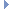OpenMM
RBTorsionForce Class Reference

This class implements an interaction between groups of four particles that varies with the torsion angle between them according to the Ryckaert-Bellemans potential. More...Inheritance diagram for RBTorsionForce:

List of all members.

## Public Member Functions

def getNumTorsions
getNumTorsions(self) -> int
addTorsion(self, particle1, particle2, particle3, particle4, c0, c1, c2, c3, c4, c5) -> int
def getTorsionParameters
Get the force field parameters for a Ryckaert-Bellemans torsion term.
def setTorsionParameters
Set the force field parameters for a Ryckaert-Bellemans torsion term.
def updateParametersInContext
Update the per-torsion parameters in a Context to match those stored in this Force object.
def usesPeriodicBoundaryConditions
usesPeriodicBoundaryConditions(self) -> bool
def __init__
__init__(self) -> RBTorsionForce __init__(self, other) -> RBTorsionForce

this

## Detailed Description

This class implements an interaction between groups of four particles that varies with the torsion angle between them according to the Ryckaert-Bellemans potential.

To use it, create an RBTorsionForce object then call addTorsion() once for each torsion. After a torsion has been added, you can modify its force field parameters by calling setTorsionParameters(). This will have no effect on Contexts that already exist unless you call updateParametersInContext().

## Constructor & Destructor Documentation

 def __init__ ( self, args )

__init__(self) -> RBTorsionForce __init__(self, other) -> RBTorsionForce

Create a RBTorsionForce.

## Member Function Documentation

 def addTorsion ( self, particle1, particle2, particle3, particle4, c0, c1, c2, c3, c4, c5 )

addTorsion(self, particle1, particle2, particle3, particle4, c0, c1, c2, c3, c4, c5) -> int

Add a Ryckaert-Bellemans torsion term to the force field.

Parameters:
 particle1 (int) the index of the first particle forming the torsion particle2 (int) the index of the second particle forming the torsion particle3 (int) the index of the third particle forming the torsion particle4 (int) the index of the fourth particle forming the torsion c0 (double) the coefficient of the constant term, measured in kJ/mol c1 (double) the coefficient of the 1st order term, measured in kJ/mol c2 (double) the coefficient of the 2nd order term, measured in kJ/mol c3 (double) the coefficient of the 3rd order term, measured in kJ/mol c4 (double) the coefficient of the 4th order term, measured in kJ/mol c5 (double) the coefficient of the 5th order term, measured in kJ/mol
Returns:
(int) the index of the torsion that was added
 def getNumTorsions ( self )

getNumTorsions(self) -> int

Get the number of Ryckaert-Bellemans torsion terms in the potential function

 def getTorsionParameters ( self, index )

Get the force field parameters for a Ryckaert-Bellemans torsion term.

Parameters:
 index (int) the index of the torsion for which to get parameters
Returns:
(int) the index of the first particle forming the torsion
(int) the index of the second particle forming the torsion
(int) the index of the third particle forming the torsion
(int) the index of the fourth particle forming the torsion
(double) the coefficient of the constant term, measured in kJ/mol
(double) the coefficient of the 1st order term, measured in kJ/mol
(double) the coefficient of the 2nd order term, measured in kJ/mol
(double) the coefficient of the 3rd order term, measured in kJ/mol
(double) the coefficient of the 4th order term, measured in kJ/mol
(double) the coefficient of the 5th order term, measured in kJ/mol
 def setTorsionParameters ( self, index, particle1, particle2, particle3, particle4, c0, c1, c2, c3, c4, c5 )

Set the force field parameters for a Ryckaert-Bellemans torsion term.

Parameters:
 index (int) the index of the torsion for which to set parameters particle1 (int) the index of the first particle forming the torsion particle2 (int) the index of the second particle forming the torsion particle3 (int) the index of the third particle forming the torsion particle4 (int) the index of the fourth particle forming the torsion c0 (double) the coefficient of the constant term, measured in kJ/mol c1 (double) the coefficient of the 1st order term, measured in kJ/mol c2 (double) the coefficient of the 2nd order term, measured in kJ/mol c3 (double) the coefficient of the 3rd order term, measured in kJ/mol c4 (double) the coefficient of the 4th order term, measured in kJ/mol c5 (double) the coefficient of the 5th order term, measured in kJ/mol
 def updateParametersInContext ( self, context )

Update the per-torsion parameters in a Context to match those stored in this Force object.

This method provides an efficient method to update certain parameters in an existing Context without needing to reinitialize it. Simply call setTorsionParameters() to modify this object's parameters, then call updateParametersInContext() to copy them over to the Context.

The only information this method updates is the values of per-torsion parameters. The set of particles involved in a torsion cannot be changed, nor can new torsions be added.

 def usesPeriodicBoundaryConditions ( self )

usesPeriodicBoundaryConditions(self) -> bool

Returns whether or not this force makes use of periodic boundary conditions.

Returns:
(bool) true if force uses PBC and false otherwise

Reimplemented from Force.

## Member Data Documentation

Reimplemented from Force.

The documentation for this class was generated from the following file:
• openmm.py